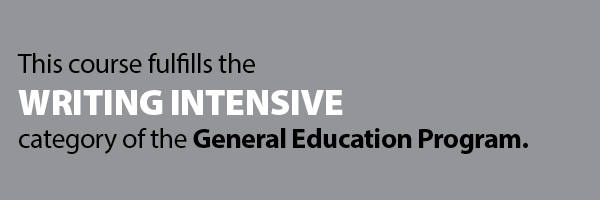Oct 15, 2019

MATH 419W - Introduction to Stochastic Mathematical Modeling [GEWI]

Models of randomness in a variety of fields: actuarial studies, economics, biology, engineering and others as appropriate for student population. Discrete time Markov chains, Poisson processes and generalizations, time series, Brownian motion, and dynamic programming. An important part of the course is an opportunity for a student to become involved in an actual modeling problem.

Credit 3 hrs May not be repeated for additional credit
Grade Mode Normal (A-F) Course Rotation Winter (odd yrs.)

Prerequisites - (MATH 223 , MATH 311W , MATH 319 , STAT 360 , or STAT 370 ) and (MATH 122 )
Restriction by Major -
Restriction by Class -Keywords: Writing Intensive (GEWI) , mathematics